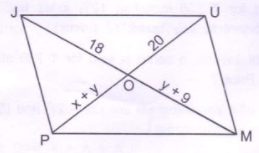# Important 4 Marks Questions for CBSE 8 Maths

Class 8th CBSE is a very important stage in the development of fundamentals of a student. As the basics of Maths and Science help students to understand complex topics which will come in 9th standard. Most of the subjects in class 8 are vast, where some of them require not only memorization but also requires skill. One such is the subject of Mathematics, which seems to be a nightmare for students and requires a lot of practice to boost confidence. So we at BYJU’S provide students of Class 8 with important 4 marks question, which can be beneficial for them in their examination.

Students preparing for CBSE Class 8 Maths Examination are advised to practice the given question for Mathematics:

Q1) Sohan wants to prepare a chart on education of children for a social work campaign. The chart is in shape of a parallelogram HEAR with HE = 5cm, EA = 6cm and $\angle R = 85^{\circ}$ .

1. Construct the parallelogram HEAR.
2. Do you think education of children is important for development of a society. Justify your statement.

Q2) Divide the sum of $\frac{7}{8}$ and $\frac{15}{24}$ by their difference. Express your answer in standard form.

Q3) The denominator of a rational number is greater than its numerator by 6. If the numerator is decreased by 1 and denominator is increased by 1, the number obtained is $\frac{1}{3}$ . Find the rational number.

Q4) PJUM is a parallelogram. Its diagonal meet at point O. Find x and y.Q5) Draw a histogram for the daily earnings of 40 drug stores in following table:

 Daily Earnings in Rupees 450-500 500-550 550-600 600-650 650-700 Number of Stores 16 10 8 4 2

Q6) Sanya took a loan of Rs. 80000 from a bank. If the rate of interest is 10% per annum, find the difference in amount she would pay after $1\frac{1}{2}$ years, if the interest is compounded:

• a) Annually
• b) Half Yearly

Q7) Sarita borrowed Rs. 18000 from Vimla at 3% per annum simple interest for 2 years. If she had borrowed this sum at 3% per annum compound interest, what extra amount would she have to pay?

Q8) Simplify and write the answer with positive exponents:

$\frac{3^{-5}\times 10^{-5}\times 125\times t^{4}}{5^{-7}\times t^{-2}}$

Q9) 500 people were stranded on an island they had enough food to last for 30 days. How long the food would last if there were 200 more people?

Q10) 6 persons could build a wall in 10 days. 3 persons left before the work started. How long it would take to build the wall now?

Q11) Draw a graph for the following table of values, with suitable scale on the axis:

 Deposit(in Rs) 1000 2000 3000 4000 5000 Simple Interest(in Rs) 80 160 240 320 400

Q12) If each edge of a cube is doubled

• a) How many times will its surface area increase?
• b) How many times will its volume increase?

Q13) A rectangular piece of paper 12 cm x 5 cm is folded without overlapping to make a cylinder of height 4 cm. Find the volume of the cylinder.

Q14) Subtract the sum of 3a + 2b – c and 5b – 6a +2ab from the sum of 9b -11ab and -4a + 21ab

Q15) In a cylindrical dome there are 16 pillars. The radius of each pillar is 35cm and height is 7m. Find the total cost of painting the curved surface area of all pillars at the rate of Rs. 8.50 per $m^{2}$ .

Q16) Water is pouring in a cuboidal reservoir at the rate of 70 litres per minute. If the volume of reservoir is 108 $m^{3}$ , find the number of hours it will take to fill the reservoir. What is the importance of saving water ?

Q17) Praful reads 15 pages of a story book in a day. Raju reads 10 pages of the same book in a day. How many days will Raju take to finish the fiction book if praful finishes in 13 days ?

Q18) Find the least number that must be added to 9911 so as to get a perfect square. Also find the square root of perfect square obtained.

Q19) Plot the distance time graph for given values:

 Distance (in km) 125 150 175 200 Time (min.) 25 30 35 40

Q20) Factorise the expressions and divide them;

$lm(l^{4} – m^{4})\div l(l^{2}+m^{2})$<

#### 1 Comment

1. Ritik singhania

Good learning